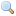# Module Nativeint

`module Nativeint: `sig` .. `end``
Processor-native integers.

This module provides operations on the type `nativeint` of signed 32-bit integers (on 32-bit platforms) or signed 64-bit integers (on 64-bit platforms). This integer type has exactly the same width as that of a `long` integer type in the C compiler. All arithmetic operations over `nativeint` are taken modulo 232 or 264 depending on the word size of the architecture.

Performance notice: values of type `nativeint` occupy more memory space than values of type `int`, and arithmetic operations on `nativeint` are generally slower than those on `int`. Use `nativeint` only when the application requires the extra bit of precision over the `int` type.

`val zero : `nativeint``
The native integer 0.
`val one : `nativeint``
The native integer 1.
`val minus_one : `nativeint``
The native integer -1.
`val neg : `nativeint -> nativeint``
Unary negation.
`val add : `nativeint -> nativeint -> nativeint``
`val sub : `nativeint -> nativeint -> nativeint``
Subtraction.
`val mul : `nativeint -> nativeint -> nativeint``
Multiplication.
`val div : `nativeint -> nativeint -> nativeint``
Integer division. Raise `Division_by_zero` if the second argument is zero. This division rounds the real quotient of its arguments towards zero, as specified for `Pervasives.(/)`.
`val rem : `nativeint -> nativeint -> nativeint``
Integer remainder. If `y` is not zero, the result of `Nativeint.rem x y` satisfies the following properties: `Nativeint.zero <= Nativeint.rem x y < Nativeint.abs y` and `x = Nativeint.add (Nativeint.mul (Nativeint.div x y) y) (Nativeint.rem x y)`. If `y = 0`, `Nativeint.rem x y` raises `Division_by_zero`.
`val succ : `nativeint -> nativeint``
Successor. `Nativeint.succ x` is `Nativeint.add x Nativeint.one`.
`val pred : `nativeint -> nativeint``
Predecessor. `Nativeint.pred x` is `Nativeint.sub x Nativeint.one`.
`val abs : `nativeint -> nativeint``
Return the absolute value of its argument.
`val size : `int``
The size in bits of a native integer. This is equal to `32` on a 32-bit platform and to `64` on a 64-bit platform.
`val max_int : `nativeint``
The greatest representable native integer, either 231 - 1 on a 32-bit platform, or 263 - 1 on a 64-bit platform.
`val min_int : `nativeint``
The greatest representable native integer, either -231 on a 32-bit platform, or -263 on a 64-bit platform.
`val logand : `nativeint -> nativeint -> nativeint``
Bitwise logical and.
`val logor : `nativeint -> nativeint -> nativeint``
Bitwise logical or.
`val logxor : `nativeint -> nativeint -> nativeint``
Bitwise logical exclusive or.
`val lognot : `nativeint -> nativeint``
Bitwise logical negation
`val shift_left : `nativeint -> int -> nativeint``
`Nativeint.shift_left x y` shifts `x` to the left by `y` bits. The result is unspecified if `y < 0` or `y >= bitsize`, where `bitsize` is `32` on a 32-bit platform and `64` on a 64-bit platform.
`val shift_right : `nativeint -> int -> nativeint``
`Nativeint.shift_right x y` shifts `x` to the right by `y` bits. This is an arithmetic shift: the sign bit of `x` is replicated and inserted in the vacated bits. The result is unspecified if `y < 0` or `y >= bitsize`.
`val shift_right_logical : `nativeint -> int -> nativeint``
`Nativeint.shift_right_logical x y` shifts `x` to the right by `y` bits. This is a logical shift: zeroes are inserted in the vacated bits regardless of the sign of `x`. The result is unspecified if `y < 0` or `y >= bitsize`.
`val of_int : `int -> nativeint``
Convert the given integer (type `int`) to a native integer (type `nativeint`).
`val to_int : `nativeint -> int``
Convert the given native integer (type `nativeint`) to an integer (type `int`). The high-order bit is lost during the conversion.
`val of_float : `float -> nativeint``
Convert the given floating-point number to a native integer, discarding the fractional part (truncate towards 0). The result of the conversion is undefined if, after truncation, the number is outside the range [`Nativeint.min_int`, `Nativeint.max_int`].
`val to_float : `nativeint -> float``
Convert the given native integer to a floating-point number.
`val of_int32 : `int32 -> nativeint``
Convert the given 32-bit integer (type `int32`) to a native integer.
`val to_int32 : `nativeint -> int32``
Convert the given native integer to a 32-bit integer (type `int32`). On 64-bit platforms, the 64-bit native integer is taken modulo 232, i.e. the top 32 bits are lost. On 32-bit platforms, the conversion is exact.
`val of_string : `string -> nativeint``
Convert the given string to a native integer. The string is read in decimal (by default) or in hexadecimal, octal or binary if the string begins with `0x`, `0o` or `0b` respectively. Raise `Failure "int_of_string"` if the given string is not a valid representation of an integer, or if the integer represented exceeds the range of integers representable in type `nativeint`.
`val to_string : `nativeint -> string``
Return the string representation of its argument, in decimal.
`type t = `nativeint` `
An alias for the type of native integers.
`val compare : `t -> t -> int``
The comparison function for native integers, with the same specification as `Pervasives.compare`. Along with the type `t`, this function `compare` allows the module `Nativeint` to be passed as argument to the functors `Set.Make` and `Map.Make`.